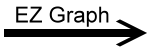﻿ EZ Graph - Tangent Function, Vertical Scaling Variable | Zona Land Education

# EZ Graph - Tangent Function, Vertical Scaling Variable

Click the 'Draw graph' button in the lower left corner of the EZ Graph app. Explanation below.

Here is the conventional notation for these tangent functions and the computer language type of notation used with EZ Graph:

 f1(x) = (a)tan(x)f1(x) = a * tan(x) f2(x) = tan(x)f2(x) = tan(x)

Notice the necessary multiplication operator (*) in the EZ Graph notation between a and tan(x)for f1(x).

Custom Search